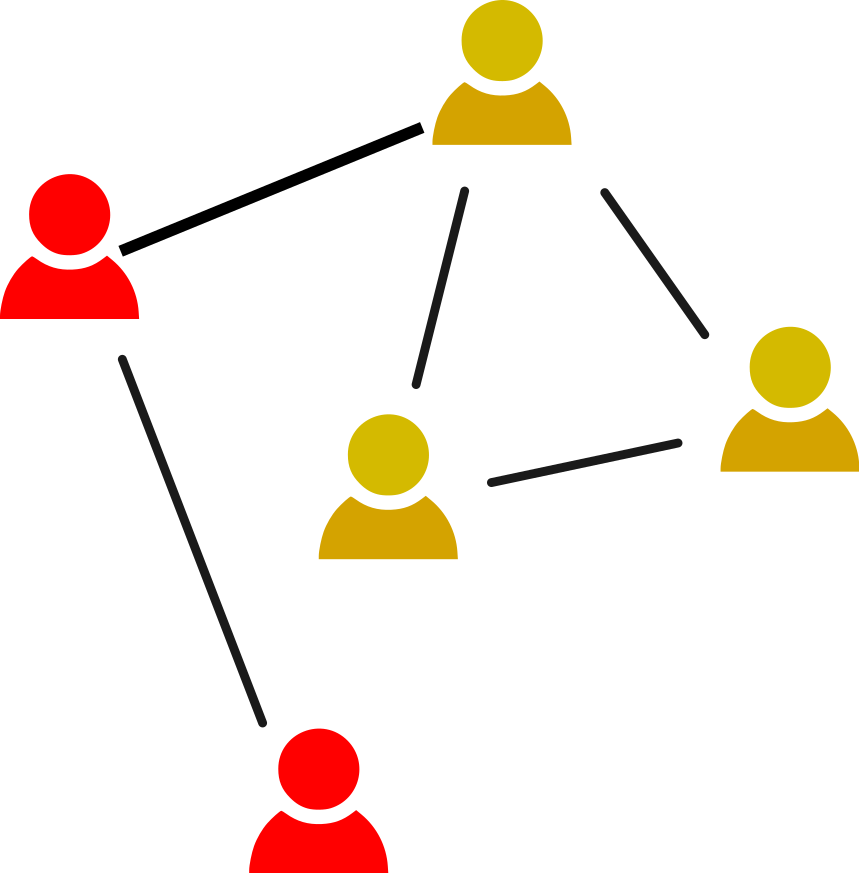### Measuring AssortativityAssortativity: A network property by which similar nodes are more likely to be connected than dissimilar nodes

Similarity is based on some node property, e.g. degree, age, political orientation, gender… Can be measured with the assortativity coefficient:

$\begin{equation*} r=\frac{\sum_c e_{cc}-\sum_c a_c b_c}{1-\sum_c a_c b_c} \end{equation*}$

• $$c$$ are the classes of nodes
• $$e_{cd}$$ is the fraction of all edges connecting nodes of class $$c$$ with nodes of class $$d$$
• $$e_{cc}$$ is the fraction of all edges connecting two nodes of class $$c$$
• $$a_c=\sum_d e_{cd}$$, and $$b_c=\sum_d e_{dc}$$ (endpoint class fractions)